### Infinity

For this quiz, we're going to start with what seems like an exceedingly simple idea: how to count things.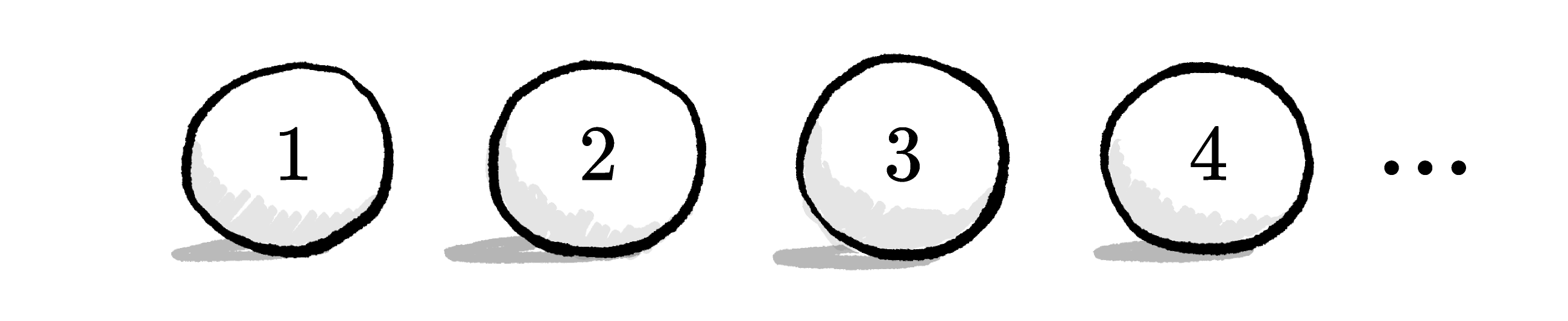However, this will soon ramp up to being able to work with infinity as a mathematical construct, not just an abstract idea. You'll be able to prove counterintuitive ideas and really get at the essence of what infinity is.

# How to Count to Infinity

Suppose you are setting a large table for $100$ people. Each person needs a plate and a napkin. You know you have exactly $100$ plates and a large number of napkins (but you're not sure how many).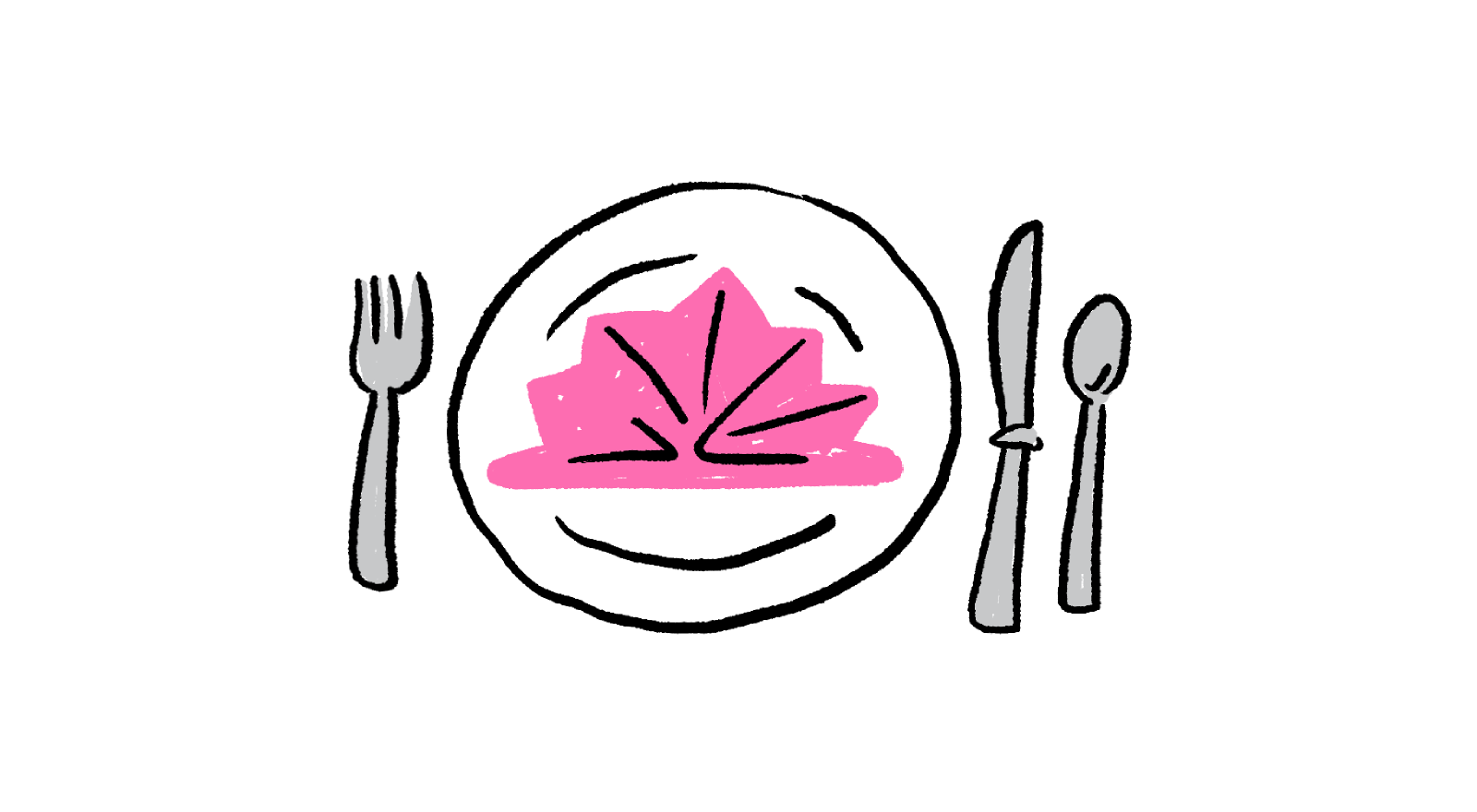You set every one of the $100$ plates out and put exactly one napkin on each of them. You then count only the leftover napkins and find that there are $32$ of them. How many napkins did you start with?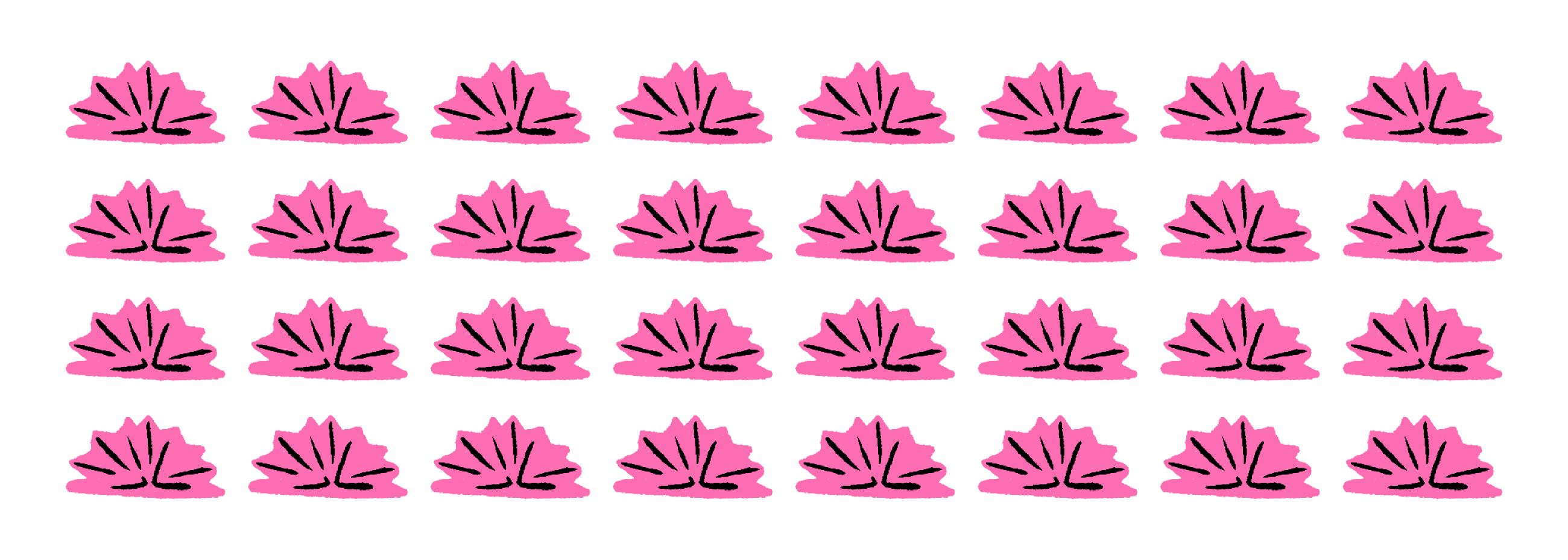# How to Count to Infinity

In the previous problem, while the $32$ napkins left over had to be "counted" in some fashion......the $100$ that matched with plates were not.

It's a subtle distinction, but comparison with another quantity is different from just counting. Imagine using a tally to make sure that all of your dishes make it through a move. You create one mark per dish when you're packing and strike through one mark per dish when you unpack. Even if you never take the time to count the total number of marks, you can figure out how many dishes you've lost (or gained) while moving.

A quick historical interlude:

The Lebombo Bone, dated to around $\num{35000}\text{ BC},$ is one of the oldest examples of comparison counting that we know of. It's a tally-mark engraved baboon femur found between South Africa and Swaziland: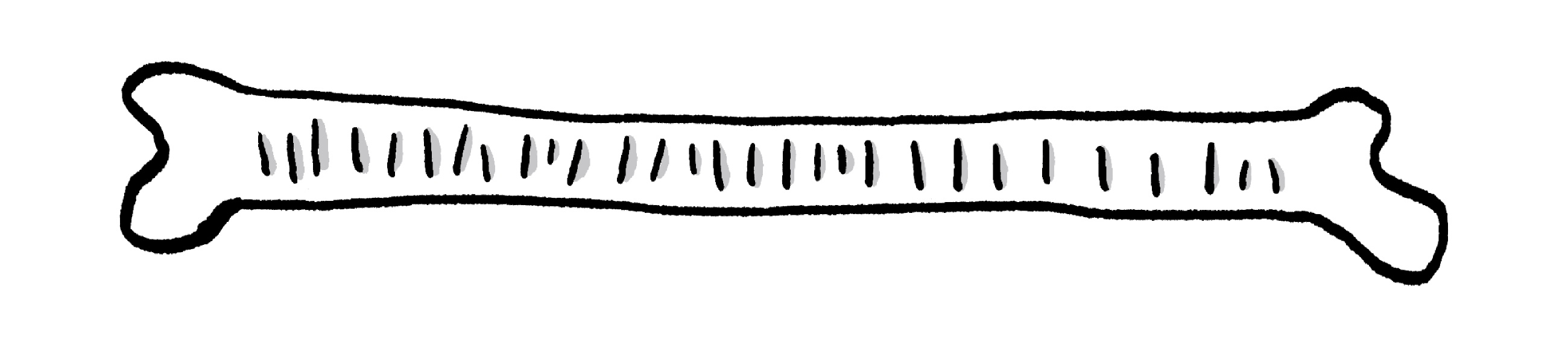There are $29$ clearly defined notches and, as $29$ corresponds to the number of days in a month on the lunar calendar, it was likely used as a "calendar stick."

# How to Count to Infinity

If the sizes of two sets of things are the same, we say they have the same cardinality.

This means, just like the plates and napkins, each value in one set has a matching value in the other set and there are no leftovers in either one.

Two sets of objects (or numbers) are said to have the same cardinality if each object in one set can be matched to one object in the other set without leaving any leftovers in either set.

In other words, if you can construct a one-to-one correspondence between the two sets, then they have the same cardinality.

Do these two finite sets have the same cardinality?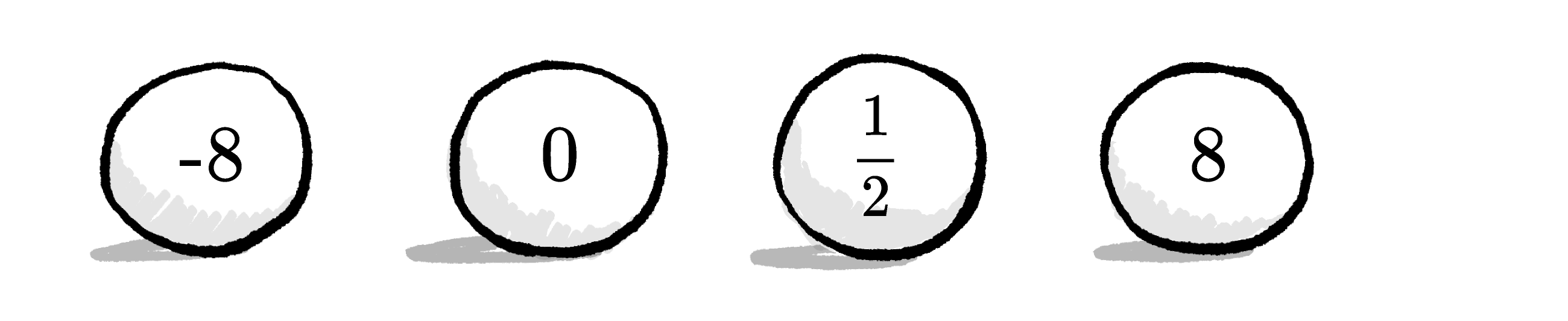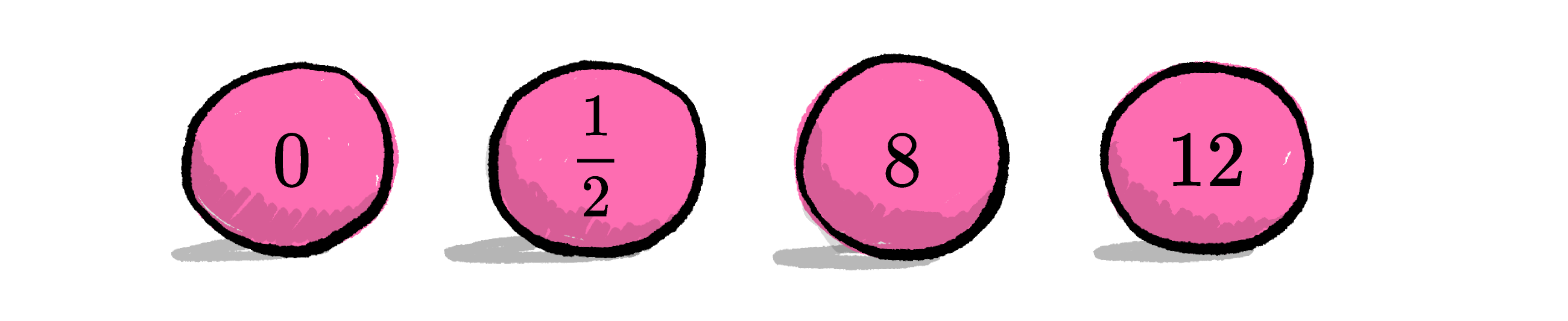# How to Count to Infinity

We can also do cardinality comparisons with infinitely large sets. Imagine an infinite row of balls marked $1, 2, 3,4,\ldots$ and so on.Next to that row, we have another row of balls marked $2, 3, 4, 5,\ldots$ and so on.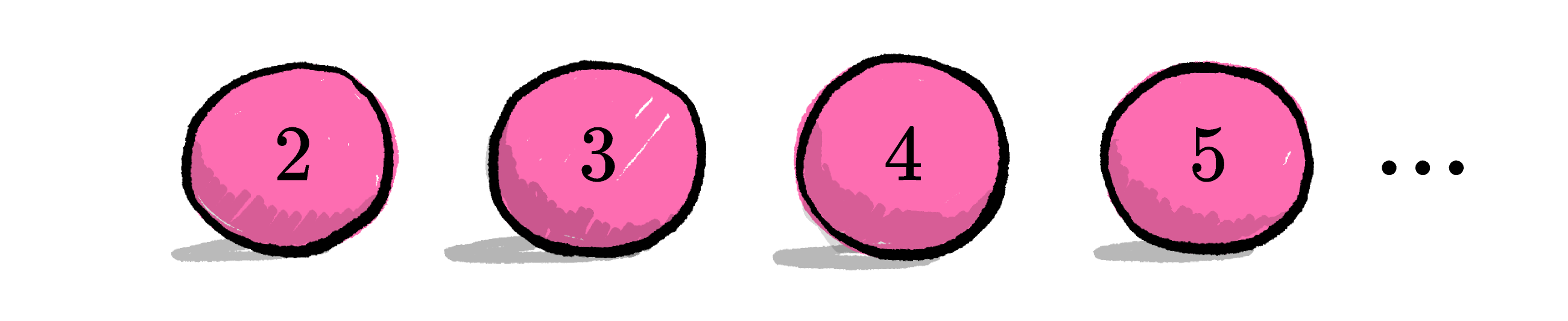Are the cardinalities of these two rows of balls the same? (Remember, this will happen if each object in one row can be matched to one object in the other without leaving leftovers — a one-to-one correspondence.)

# How to Count to Infinity

Suppose we have the $1, 2, 3, \ldots$ to infinity row of balls again......and we have a row $1, 2, 3,\ldots$ that stops at $\num{1000000}.$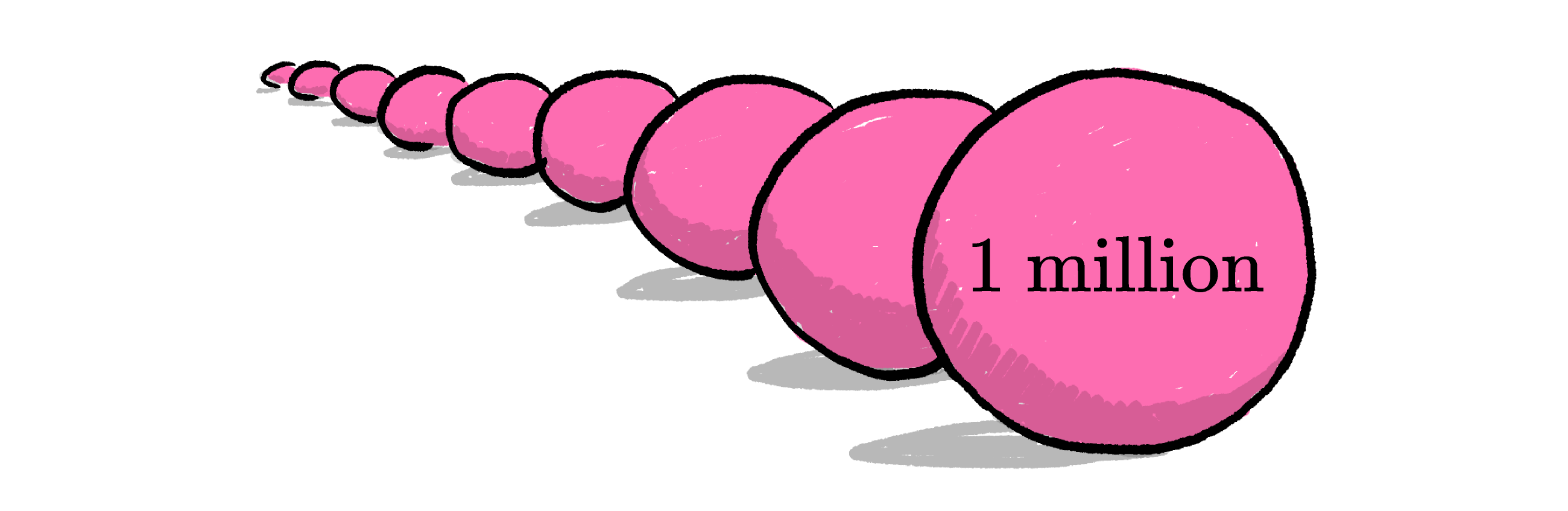Are the cardinalities the same?

# How to Count to Infinity

Compare $1, 2, 3, 4, \ldots$with the squares of all positive integers: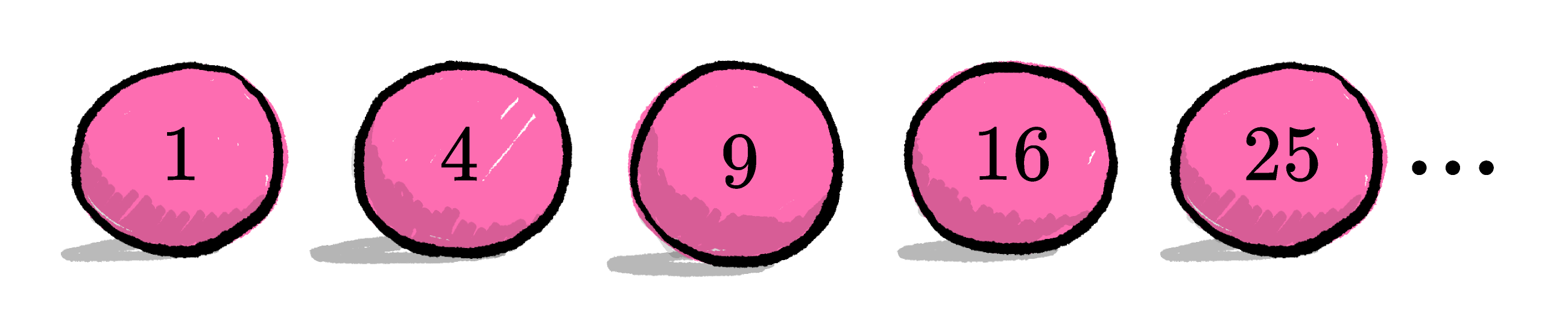Are the cardinalities the same?

# How to Count to Infinity

Let's try one more infinite match; this time we'll establish ahead of time that the cardinalities are the same and you just need to find a method of matching.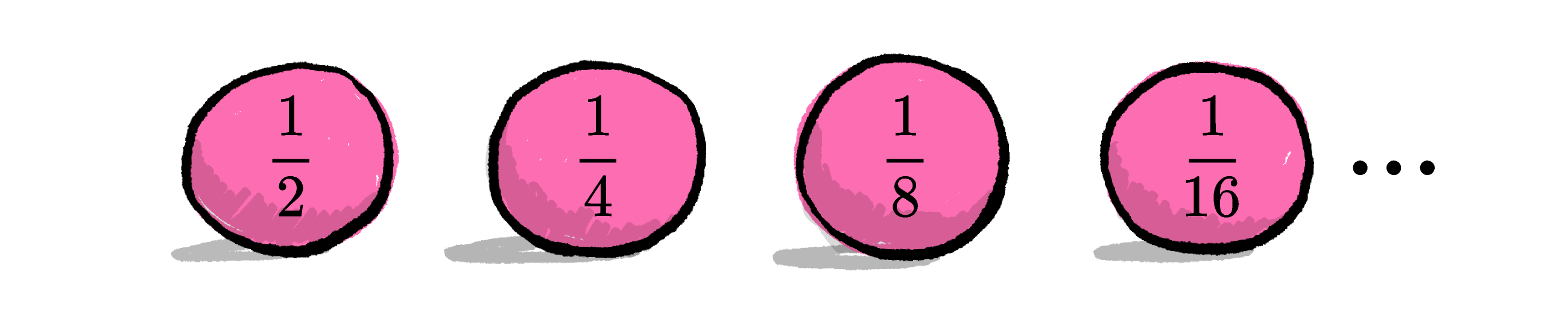If you pick a value $x$ from the first row, what can the corresponding value from the second be? (Remember, for this to work, every value from the first row has a match in the second, with no terms left out of either row.)

# How to Count to Infinity

At this point, it may appear to you that all infinite sets have the same cardinality. While the theory of infinity was being developed, this was a major open question.It turns out that there are other sizes of infinity, which we will prove later in this course!

# How to Count to Infinity

×